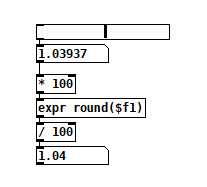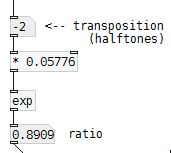• ### BPM/Pitch calculator

BPMcalculator.pd

Messing about with this simple patch to calculate BPM change when something is slowed down two semitones and I wondered - how would I find the most whole numbered/least amount of decimal places change/ratio?

I think this is probably a basic maths question, but I have no clue how to approach it. Thanks!

• Posts 7 | Views 672
• Thanks - but the maths help I need is how to find ratios between the bpm changes.

• @lead A semitone is 1/12 of an octave, so if you raise the pitch by a semitone, you're raising the tempo by 2 to the power of 1/12 and would multiply the tempo by that.
So if you lower the pitch by 2 semitones you would divide the tempo by 2 to the power of 2/12
The tempo ratio (old to new) would be 1 : (1 / 2 to the power of 0.166666667)
The tempo ratio (new to old) would be (1 / 2^0.166666667) : 1
As sure as I can be...

You can do the math in your patch using [pow]
David.

• Have you had a look at the patch I posted? I think that’s what I did!

I suppose I’m looking for the most ‘harmonic’ ratios. Would be useful going forward too I suppose when working with anharmonic spectral stuff.

• @lead I'm not sure what you're looking for, can you give us some numeric examples? If you simply want to reduce the precision of a float to some number of decimal places, you could do it this way:• @lead The ratios will be constant for semi-tones regardless of BPM (the "ratio" thing).
Sorry, I didn't see your patch (I always miss them when they are at the top of the post).
Try this........ bpm_ratio.pd
The power needs to stay positive and then is multiplied for "up" and divided for "down".
The ratios you get will keep you in tune while trying to get integers will not (except for octaves of course)..
If you want to detune then remove [int].

Screenshot below is a simpler patch but very slightly less accurate... taken from G09.pitchshift in the Pd examples.....
David.Posts 7 | Views 672
Internal error.

Oops! Looks like something went wrong!